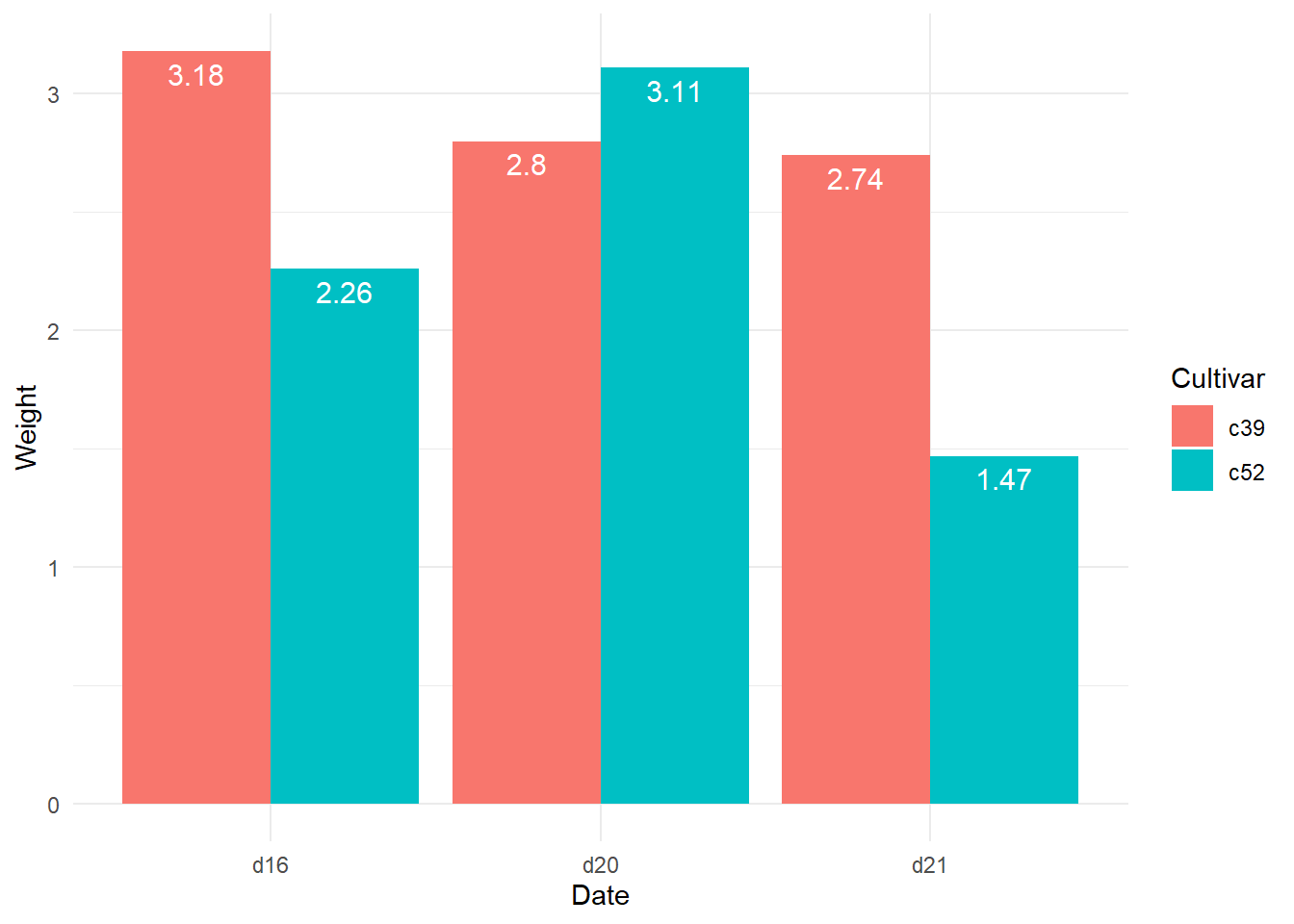# Chapter 3 ggplot2_Exercise5

## 3.1 Exercise 5-1

``````library(gcookbook)
library(tidyverse)
library(dplyr)
library(ggplot2)
ggplot(heightweight, aes(x=ageYear, y=heightIn, size=weightLb, color=sex))+geom_point()``````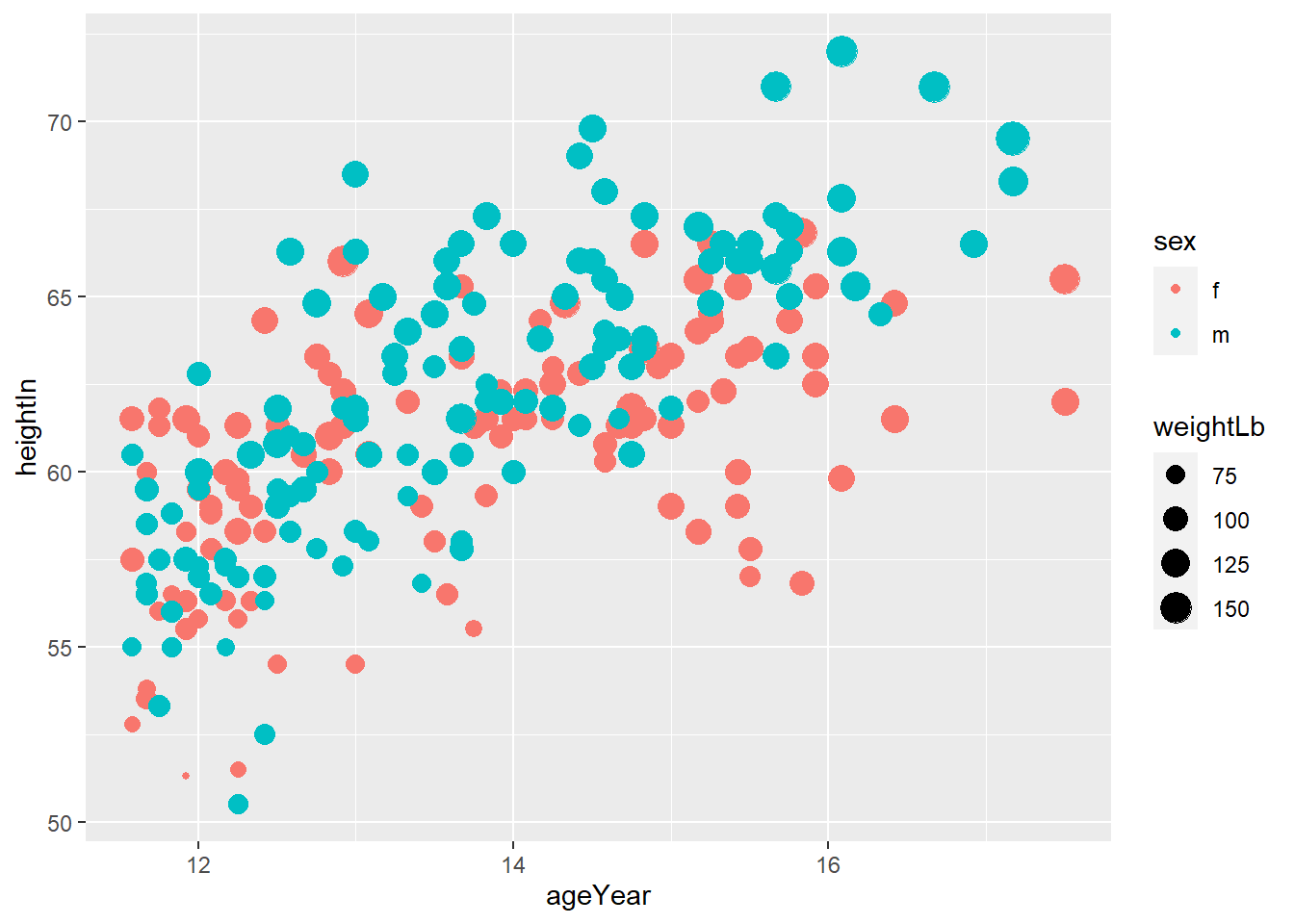``ggplot(heightweight, aes(x=ageYear, y=heightIn, size=weightLb, color=sex))+geom_point(alpha=0.3)``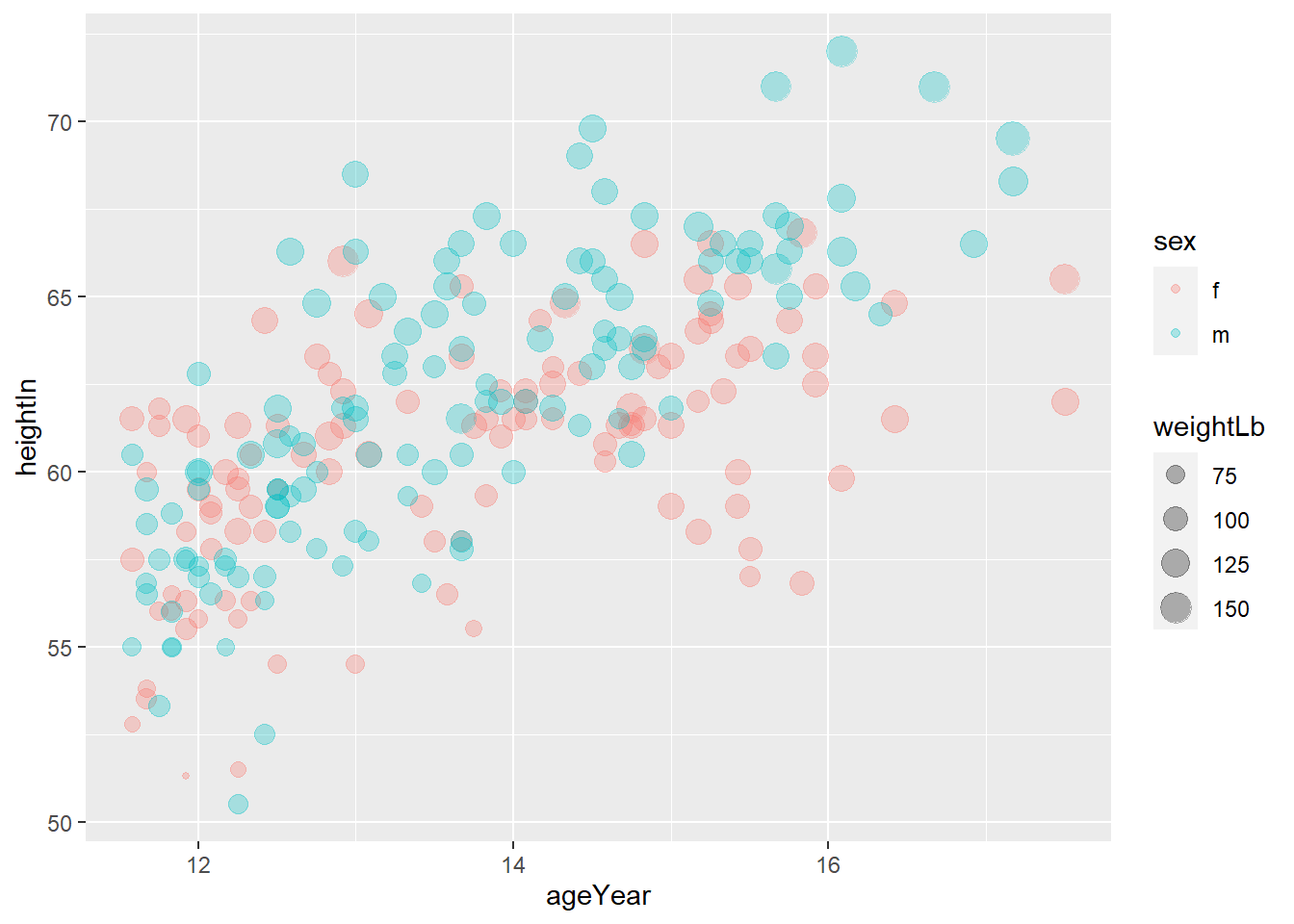``````ggplot(heightweight, aes(x=ageYear, y=heightIn, size=weightLb, color=sex))+geom_point(alpha=0.3) +
labs(title="Height and weight of school children",
subtitle="Height vs Weight",
caption="Source: heightweight",
x="Age (year)",
y="Height (inches)",
size="Weight(Lb)",
color="Gender")``````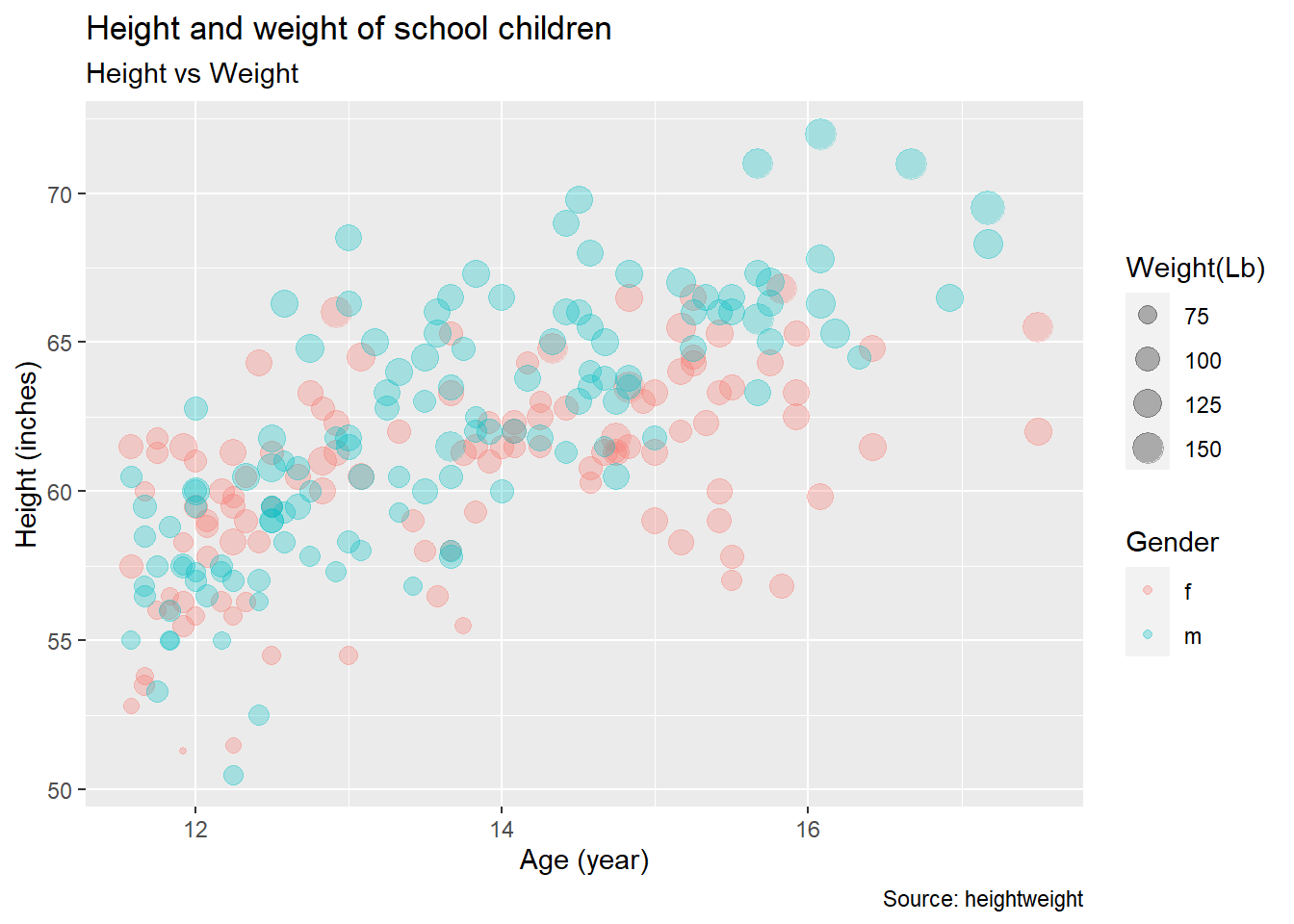``````ggplot(heightweight, aes(x=ageYear, y=heightIn, size=weightLb, color=sex))+geom_point(alpha=0.3) +
labs(title="Height and weight of school children",
subtitle="Height vs Weight",
caption="Source: heightweight",
x="Age (year)",
y="Height (inches)",
size="Weight(Lb)",
color="Gender") + theme_classic()``````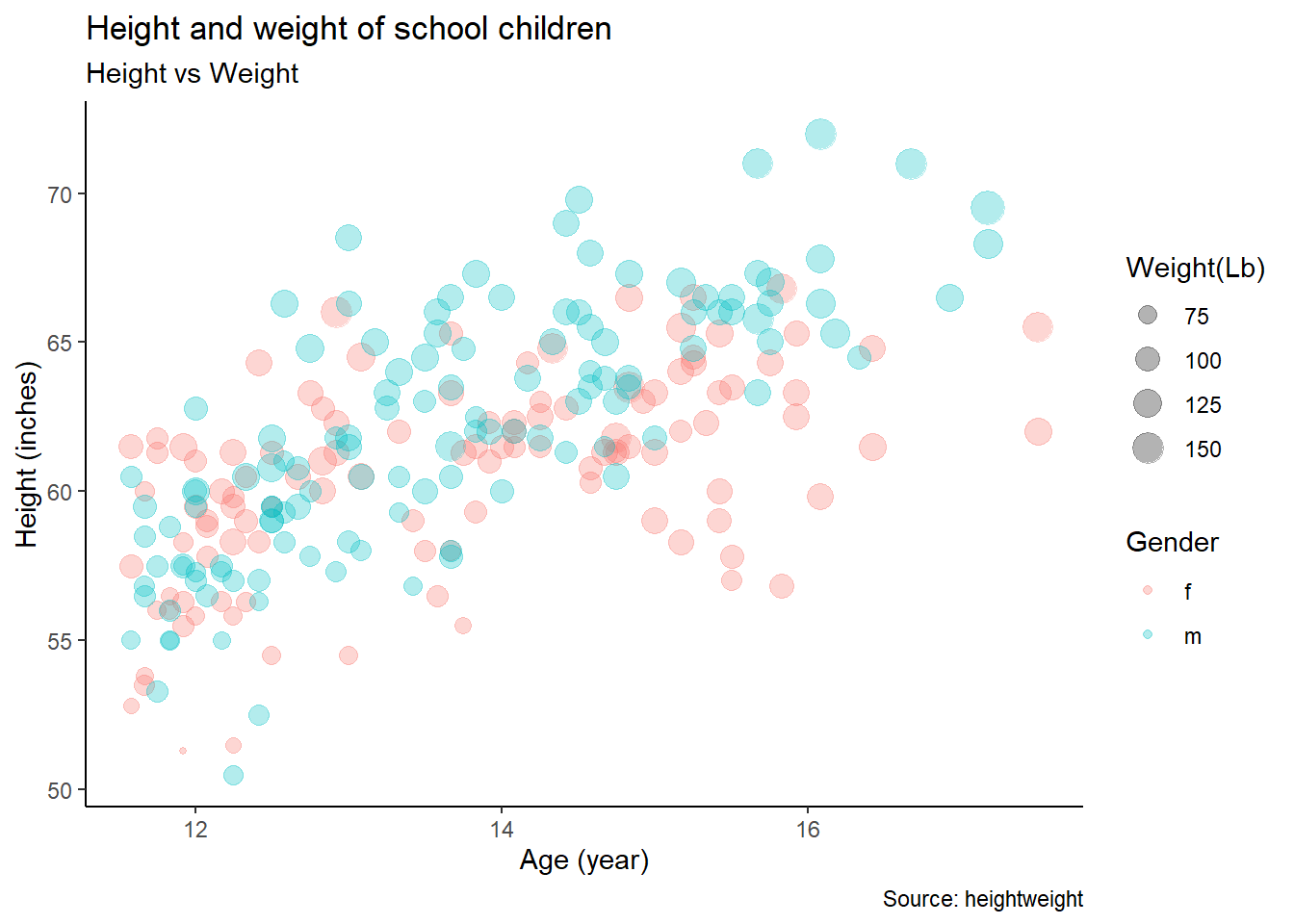## 3.2 Exercise 5-2

``ggplot(heightweight, aes(x=heightIn)) + geom_histogram()``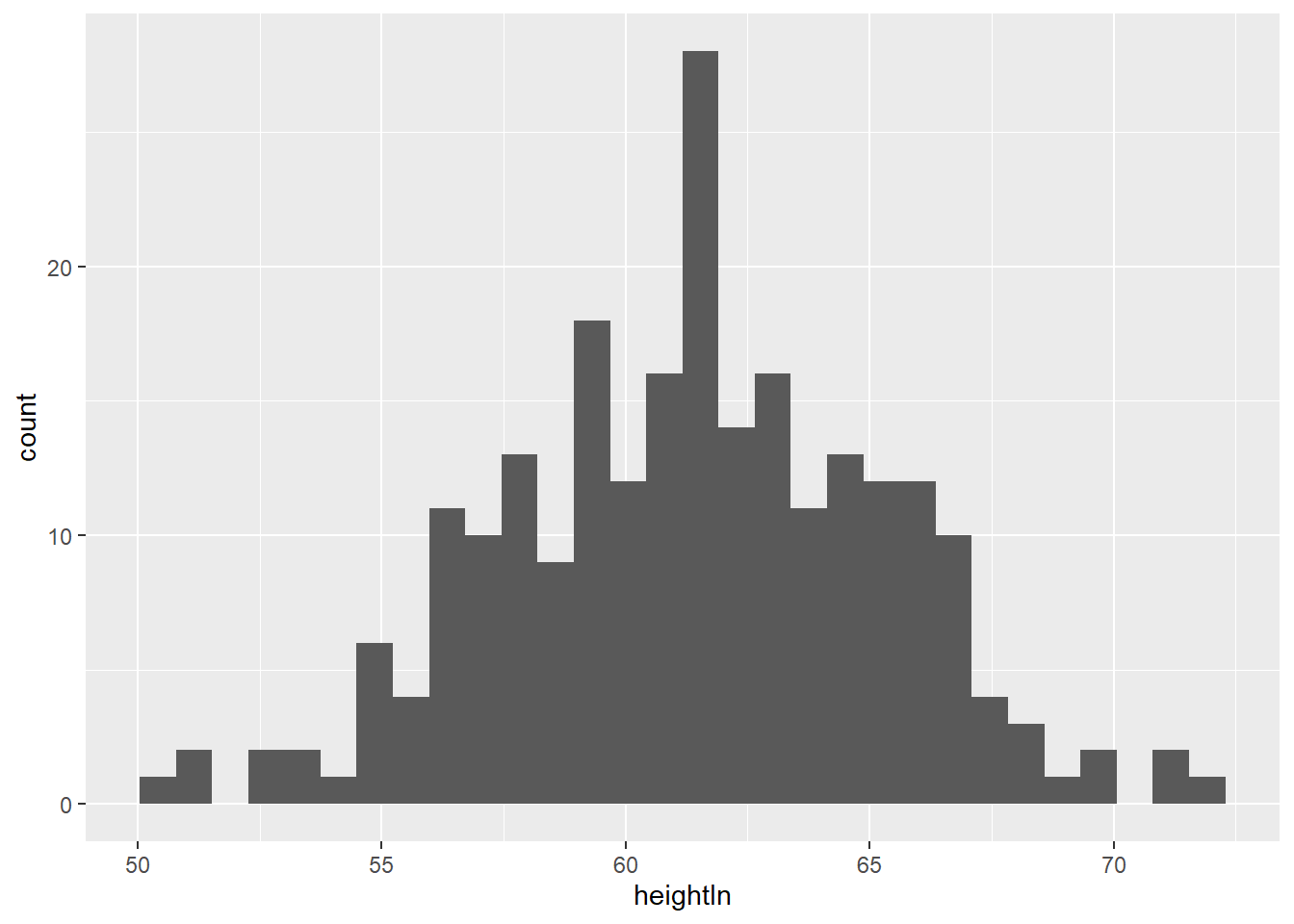``ggplot(heightweight, aes(x=weightLb, fill=sex)) + geom_histogram()``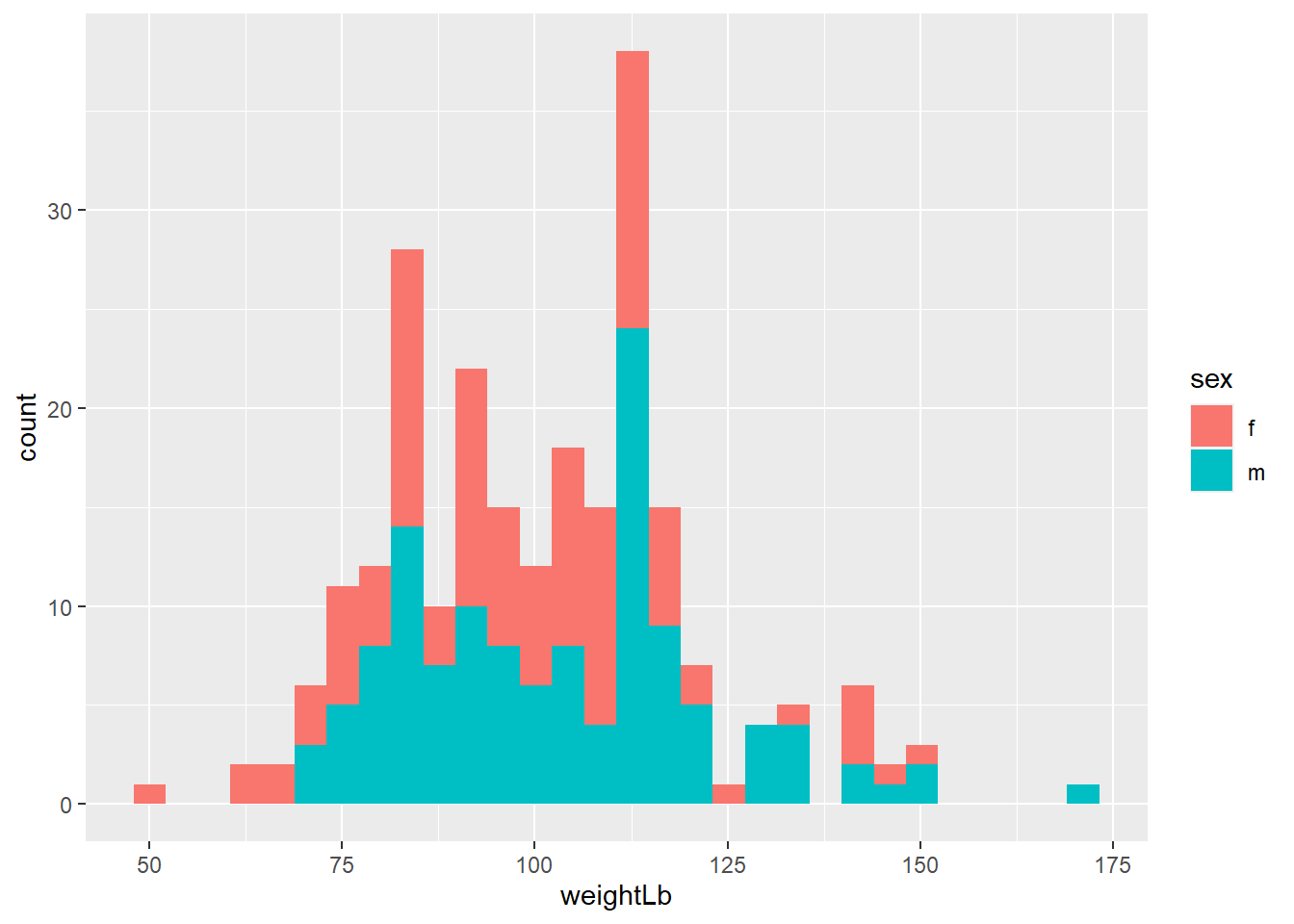``````ggplot(heightweight, aes(x=weightLb, fill=sex)) +
geom_histogram(alpha=0.4) +
scale_fill_manual(values=c("orange", "yellow"))``````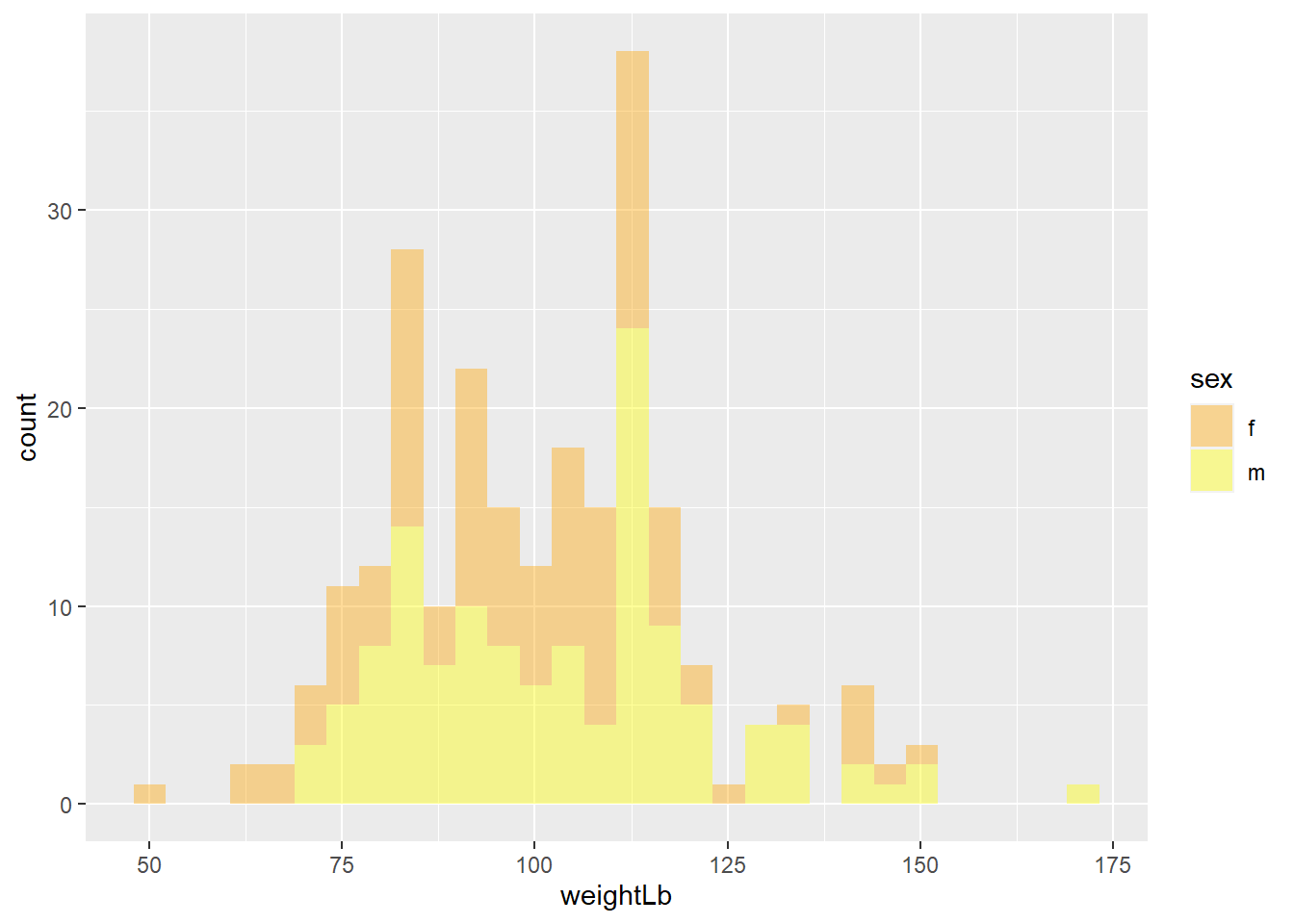``````ggplot(heightweight, aes(x=weightLb, fill=sex)) +
geom_histogram(alpha=0.4) +
scale_fill_manual(values=c("orange", "yellow")) +
labs(title = "A histogram of the weight of school children",
subtitle = "By gender",
x="WeightLb",
y="Count",
fill="Gender") + theme_minimal()``````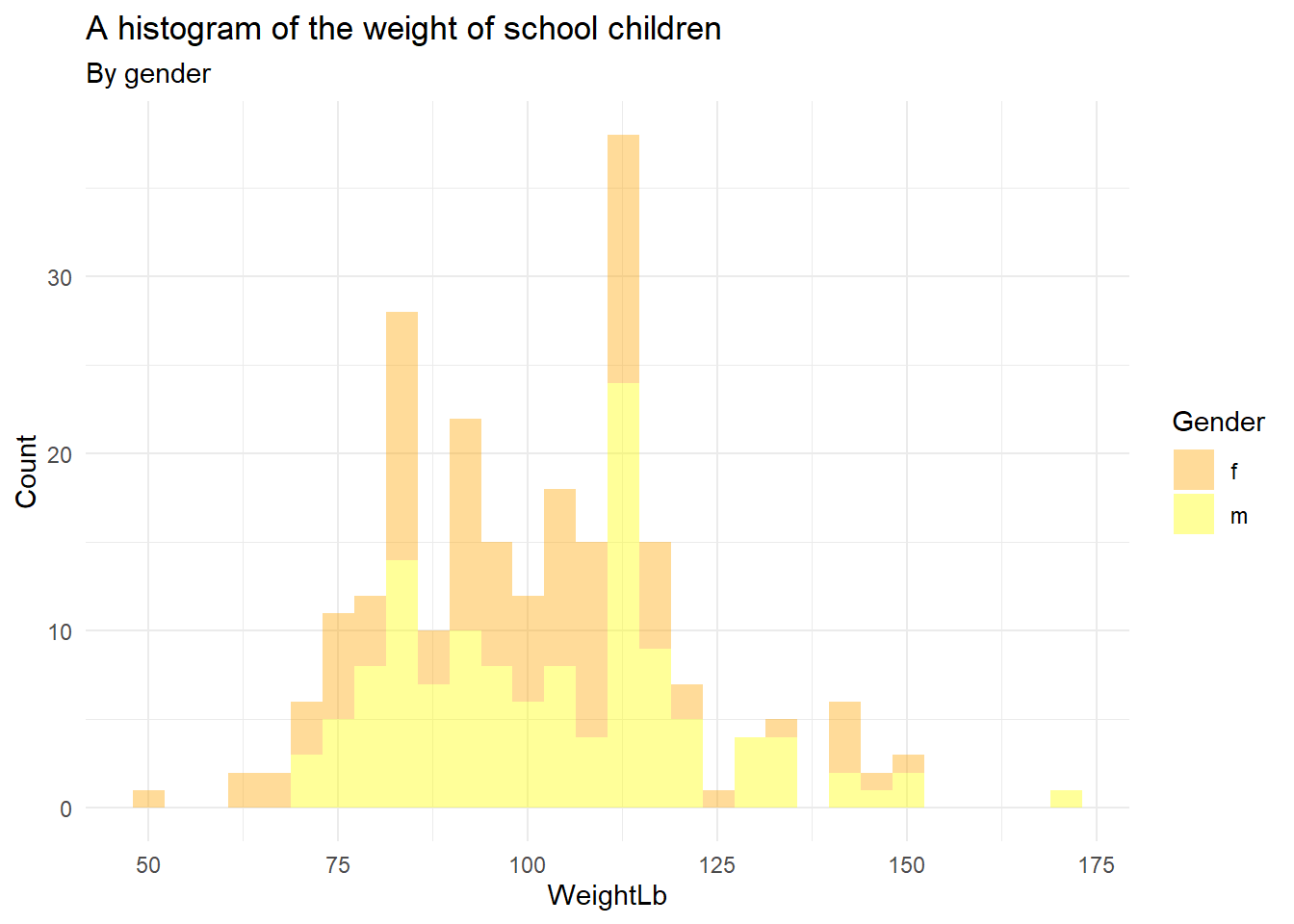## 3.3 Exercise 5-3

``````ggplot(mpg, aes(x=hwy, fill=drv)) +
geom_histogram(alpha=0.5) +
facet_grid(vars(drv)) +
labs(title= "Histogram using facet_grid()",
subtitle= "Histogram of Highway Mile Per Gallon",
caption= "Source: mpg") + theme_minimal()``````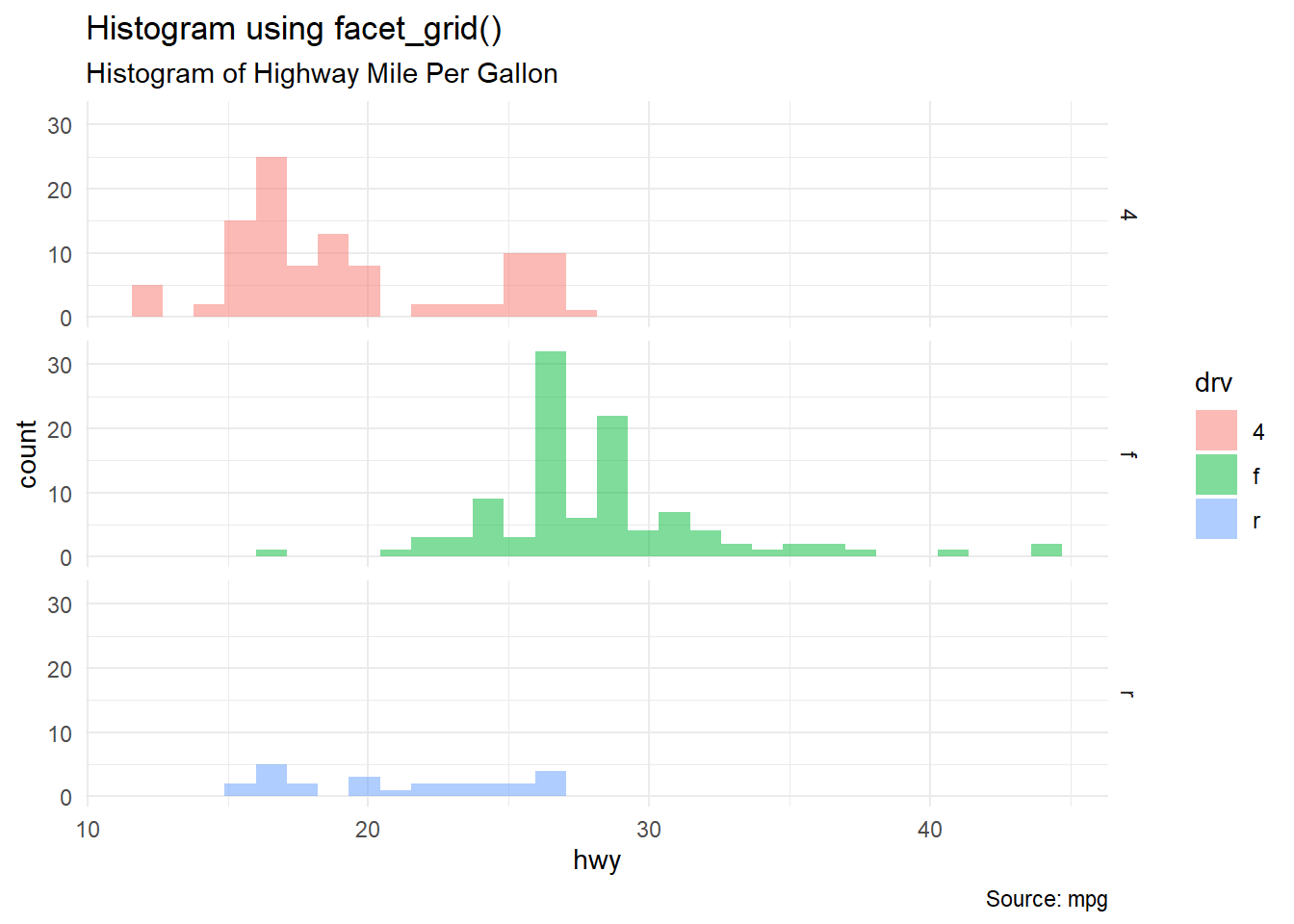## 3.4 Exercise 5-4

``````ggplot(midwest, aes(x = area, y = poptotal)) +
geom_point(alpha=0.4, aes(size = popdensity, color = state)) +
geom_smooth(se=FALSE) +
xlim(c(0, 0.1)) +
ylim(c(0, 500000)) +
labs(title = "Scatterplot",
subtitle = "Area Vs Population",
x = "Area",
y = "Population",
caption = "midwest") +
theme_classic()``````
``## Warning: Removed 15 rows containing non-finite values (stat_smooth).``
``## Warning: Removed 15 rows containing missing values (geom_point).``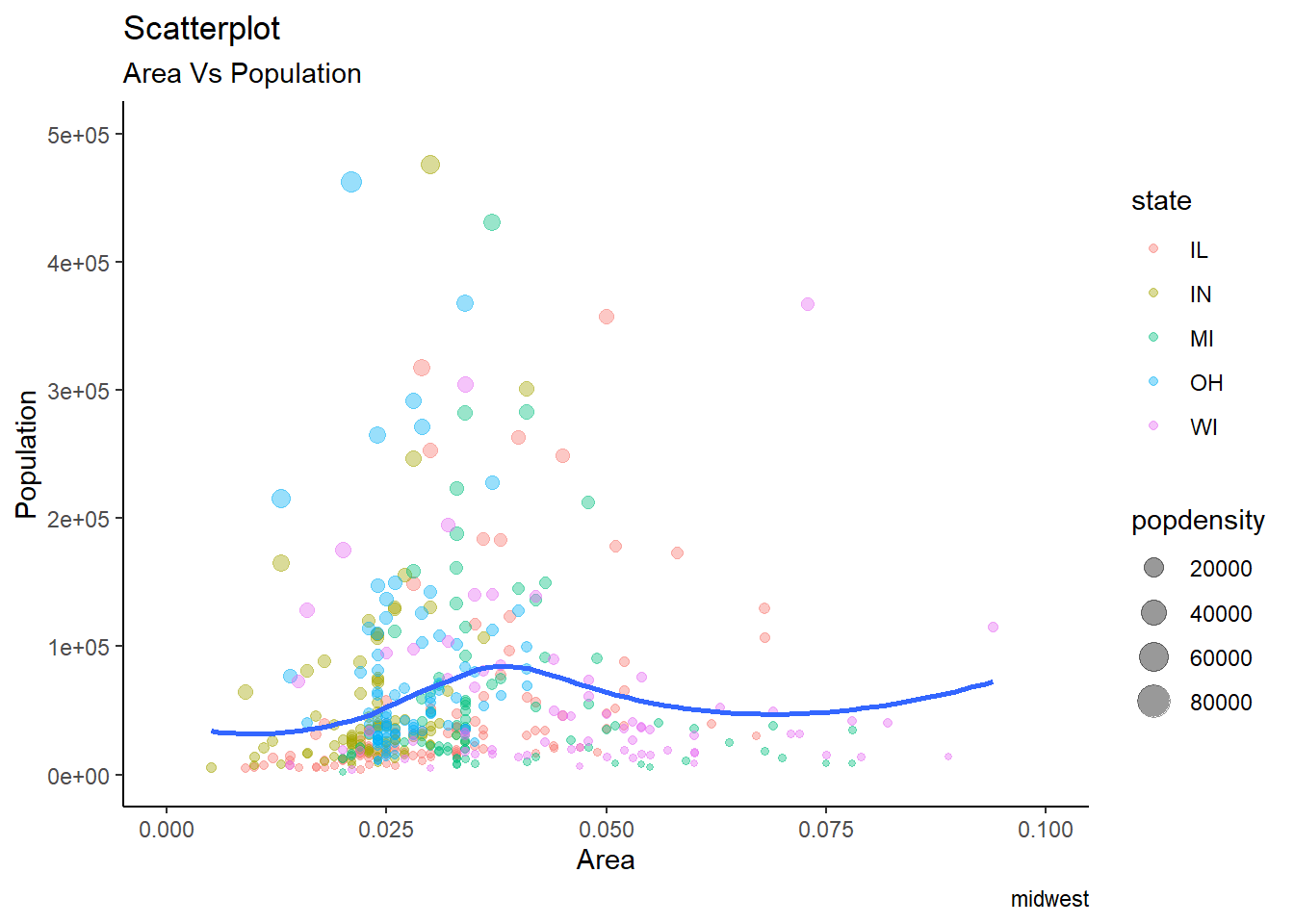## 3.5 Exercise 5-5

``````ggplot(iris, aes(x=Sepal.Length, y=Sepal.Width, shape=Species, color=Species)) +
geom_point(alpha=0.5, size=6) +
theme_minimal() +
labs(title = "Scatterplot",
subtitle = "Sepal.Length Vs Sepal.Width",
caption = "Source: iris")``````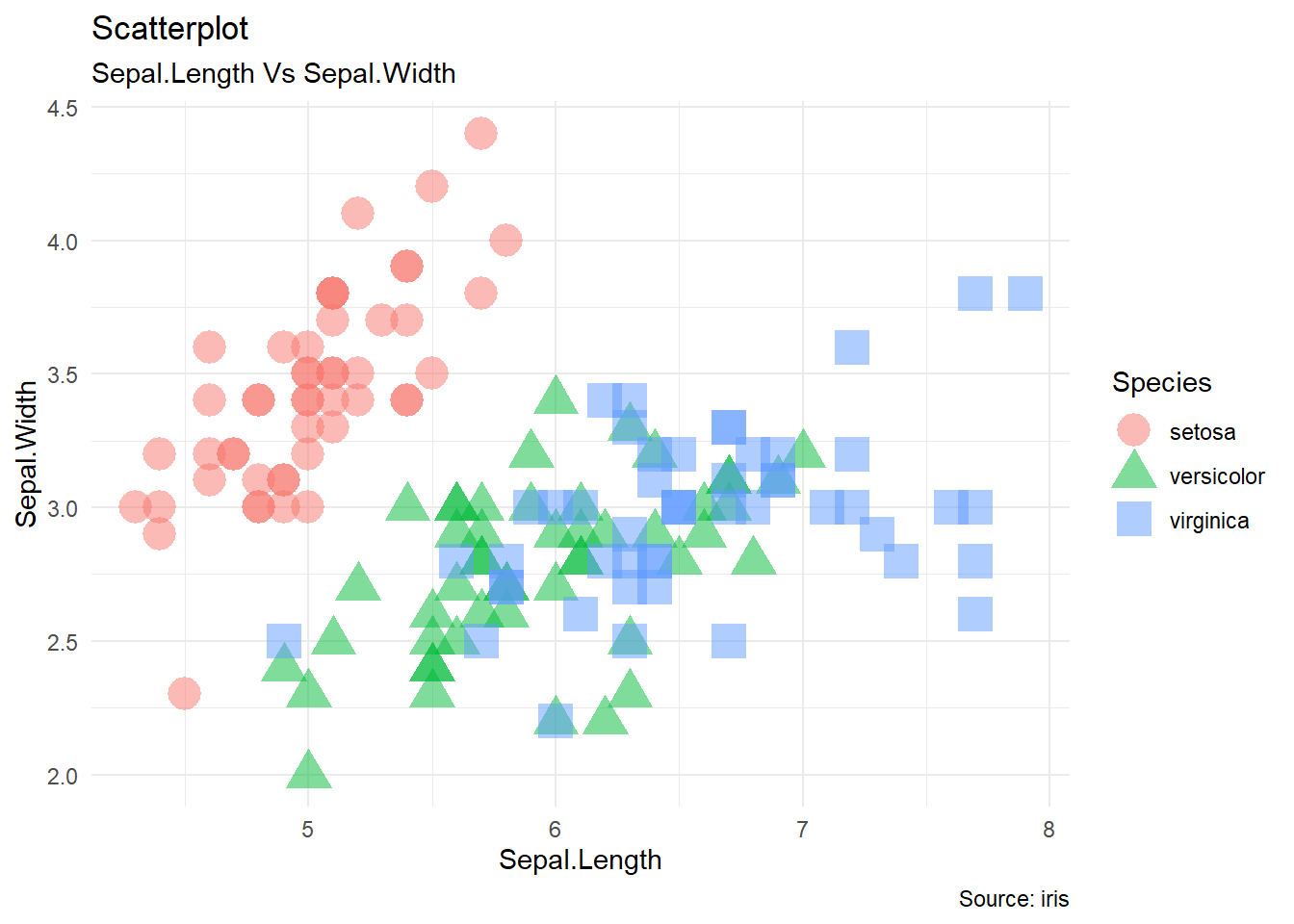## 3.6 Exercise 5-6

``````ggplot(heightweight, aes(x=heightIn, y=weightLb, color=sex)) +
geom_point(alpha=0.5, size=3) +
geom_smooth(se=FALSE, method="lm") +
theme_classic() +
labs(title = "Scatterplot",
subtitle = "Weight Vs Height",
caption = "Source: heightweight")``````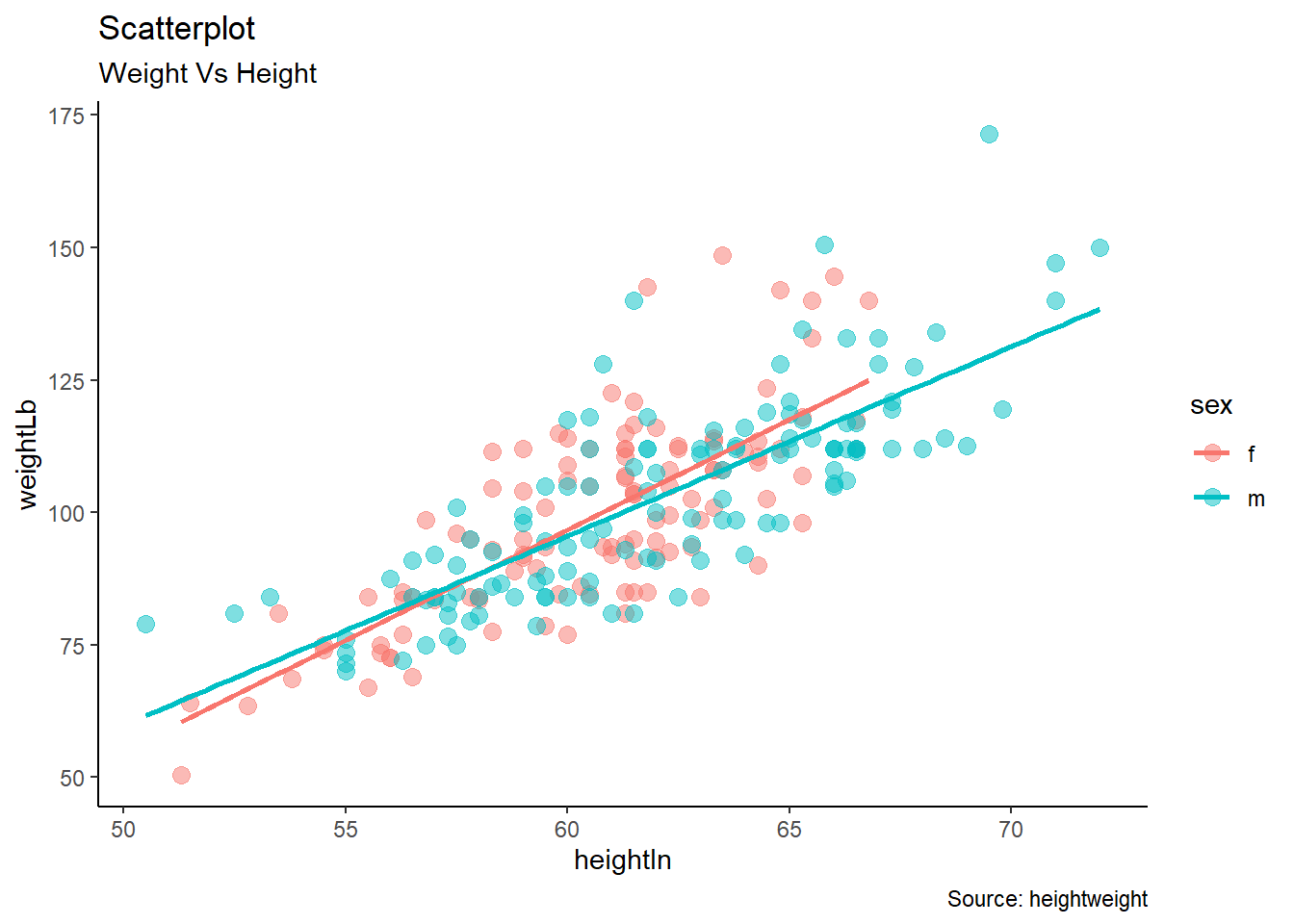## 3.7 Exercise 5-7

``````ggplot(mpg, aes(x=manufacturer, fill=class)) +
geom_bar(width = 0.5) +
theme_minimal() +
labs(title = "Barplot",
subtitle = "Manufacturer across Vehicle Classes") +
scale_fill_brewer(palette = "Spectral") +
theme(axis.text.x = element_text(angle = 65))``````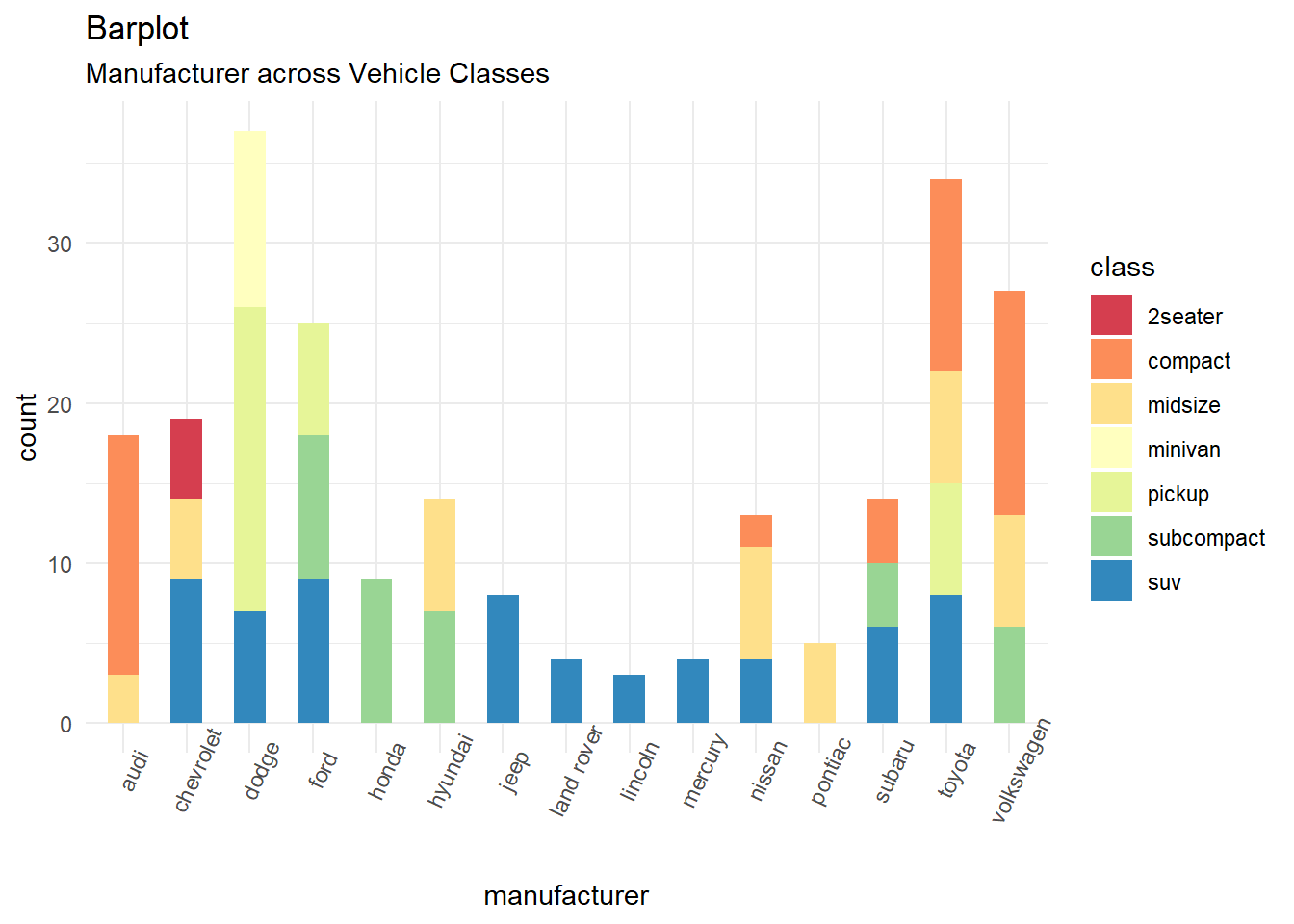## 3.8 Exercise 5-8

``````ggplot(cabbage_exp, aes(x=Date, y=Weight, fill=Cultivar)) +
geom_bar(stat='identity', position="dodge") +
geom_text(aes(label = Weight), colour = "white", size = 4, vjust = 1.5, position = position_dodge(.9)) +
theme_minimal()``````